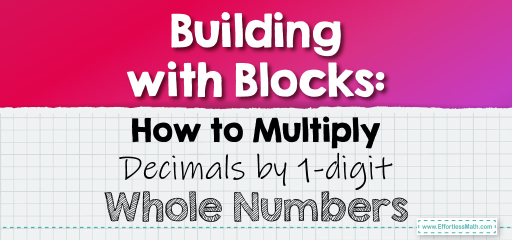# Building with Blocks: How to Multiply Decimals by 1-digit Whole Numbers

Using visual aids like blocks can make understanding mathematical concepts, especially decimals, much more intuitive. When multiplying a decimal by a 1-digit whole number, envisioning blocks can help grasp the concept better. Let's dive into how to approach this using blocks.

Visualizing with Blocks:

Imagine each block represents a unit. When we talk about decimals, we can think of them as parts of a block. For instance, if a block represents $$1$$, then $$0.1$$ would be a tenth of that block.## Multiplying a Decimal by a 1-digit Whole Number Using Blocks

### Example 1:

Multiply $$0.2$$ by $$3$$.

Solution Process:

1. Visualize $$0.2$$ as a fifth of a block.

2. If you have three such fifths, you essentially have $$3 \times 0.2$$ blocks.

Using blocks, you can see that $$0.2$$ multiplied by $$3$$ gives $$0.6$$, which is a little over half a block.

The Absolute Best Book for 5th Grade Students

### Example 2:

Multiply $$0.5$$ by $$4$$.

Solution Process:

1. Visualize $$0.5$$ as half of a block.

2. If you have four of these halves, you have $$4 \times 0.5$$ blocks.

Using blocks, you can see that $$0.5$$ multiplied by $$4$$ gives $$2$$, which is two full blocks.

Using blocks to visualize the multiplication of decimals by whole numbers provides a tangible way to understand the concept. It bridges the gap between abstract numbers and real-world representations, making the learning process engaging and effective. Whether you’re a student, teacher, or someone looking to understand decimals better, using blocks as a visual aid can be a game-changer. So, the next time you encounter a decimal multiplication problem, think in blocks!

### Practice Questions:

1. Visualize $$0.3$$ multiplied by $$5$$ using blocks.

2. How many blocks represent $$0.4$$ multiplied by $$2$$?

3. If you multiply $$0.1$$ by $$7$$, how much of a block will you have?

4. Visualize $$0.6$$ multiplied by $$3$$ using blocks.

5. How many blocks represent $$0.7$$ multiplied by $$4$$?

A Perfect Book for Grade 5 Math Word Problems!

1. $$0.3 \times 5 = 1.5$$ (One and a half blocks)

2. $$0.4 \times 2 = 0.8$$ (Almost a full block, but missing a fifth)

3. $$0.1 \times 7 = 0.7$$ (Seven-tenths of a block)

4. $$0.6 \times 3 = 1.8$$ (One full block and four-fifths of another block)

5. $$0.7 \times 4 = 2.8$$ (Two full blocks and four-fifths of another block)

The Best Math Books for Elementary Students

### What people say about "Building with Blocks: How to Multiply Decimals by 1-digit Whole Numbers - Effortless Math: We Help Students Learn to LOVE Mathematics"?

No one replied yet.

X
51% OFF

Limited time only!

Save Over 51%

SAVE $15 It was$29.99 now it is \$14.99